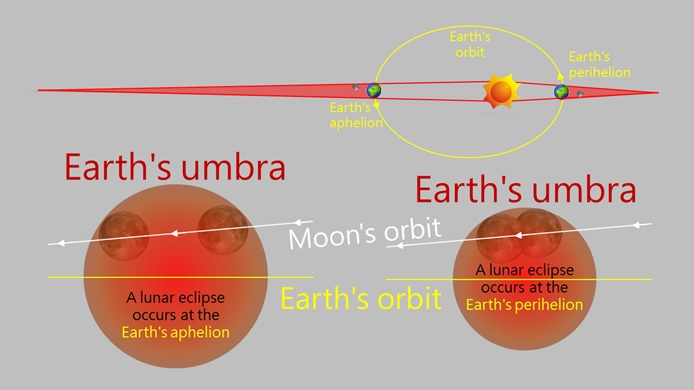# No lunar eclipses are identicalEducational Resources

No lunar eclipses are identical

The duration of each lunar eclipse differs. What factors are at play? We will make use of the astronomical concepts to unveil the phenomenon.

Both the Earth's orbit around the Sun and the Moon's orbit around the Earth are elliptical in shape (upper part of Figure 1). The two orbits are almost on the same plane with a small inclination of about 5 degrees. Such an inclination gives rise to two intersecting points called lunar nodes. During full moon, the closer the Moon gets near the lunar nodes, the more likely a lunar eclipse will occur.　An eclipse will occur when the Moon completely or partially passes through the umbra or total shadow of the Earth (detailed explanation can be found at “Lunar Eclipse”). If the Moon becomes completely immersed into the umbra, a total eclipse will occur, whereas if only a portion of the Moon falls into the umbra, there will be a partial lunar eclipse.

The first factor that affects the length of a lunar eclipse is the distance between the path of lunar eclipse and the Earth's umbra. The lower part of Figure 1 shows two typical moon's paths relative to the center of the Earth's umbra during a lunar eclipse. The right one is a case of shorter eclipse duration with the Moon skirting the outer rim of the Earth's umbra when the Moon is farther away from the lunar nodes, against the case of longer duration on the left with the Moon moving close to the center of the Earth's umbra when the Moon is located near a lunar node. In other words, the closer the Moon to a lunar node, the longer will be the duration of the eclipse.Figure 1

The second factor is the size of the Earth's umbra. If the Earth is at its perihelion (the point closest to the Sun), its umbral shadow on the Moon would be smaller and hence a shorter eclipse duration (Figure 2, right). On the contrary, larger umbra and longer eclipse duration can be expected when the Earth is near its aphelion (the point farthest from the Sun) (Figure 2, left). Thus, assuming that the Moon keeps a constant distance from the Earth, the farther the Earth from the Sun, the longer will be the length of the eclipse.Figure 2

The third factor is the apparent size of the Moon. When the Moon is at its perigee (the point closest to the Earth), it is visually bigger than usual from the Earth and it will pass through the Earth's umbral shadow at a relatively shorter duration (Figure 3, right). The reverse situation will happen when the Moon is at its apogee (the point farthest from the Earth) (Figure 3, left). As such, the farther the Moon from the Earth, the longer will be the duration of the eclipse.Figure 3

(For the purpose of illustration, all diagrams are not drawn to scale.)